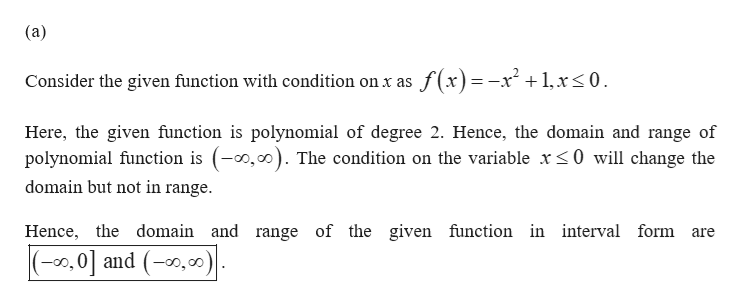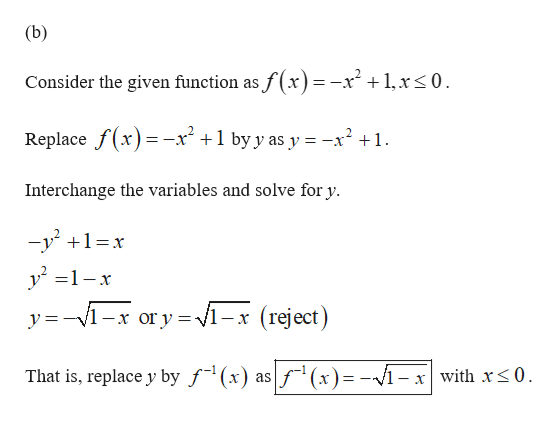# For Exercise, a.Write the domain and range of f in interval notation.b.Write an equation of the inverse function.c. Write the domain and range of f−1 in interval notation.f(x) = −x^2 + 1, x ≤ 0

Question
16 views

For Exercise,

a.Write the domain and range of f in interval notation.

b.Write an equation of the inverse function.

c. Write the domain and range of f−1 in interval notation.

f(x) = −x^2 + 1, x ≤ 0

check_circle

Step 1help_outlineImage Transcriptionclose(a) Consider the given function with condition on x as f(x)=-x² +1,r<0. Here, the given function is polynomial of degree 2. Hence, the domain and range of polynomial function is (-0,00). The condition on the variable x<0 will change the domain but not in range. Hence, the domain and range of the given function in interval form are -00,0| and (-0,00) fullscreen
Step 2help_outlineImage Transcriptionclose(b) Consider the given function as f(x)=-x² + 1,x<0. %3D Replace f(x)=-x² +1 by y as y = -x² +1. Interchange the variables and solve for y. -y +1=x y =1-x y=-V1-x or y =V1-x (reject) f"'(x)= -Vi- x with x<0. That is, replace y by ƒ¯(x) as fullscreen

### Want to see the full answer?

See Solution

#### Want to see this answer and more?

Solutions are written by subject experts who are available 24/7. Questions are typically answered within 1 hour.*

See Solution
*Response times may vary by subject and question.
Tagged in
MathAlgebra

### Other